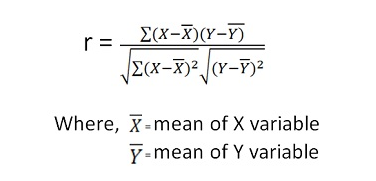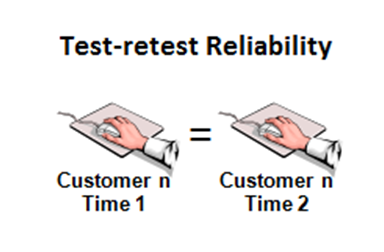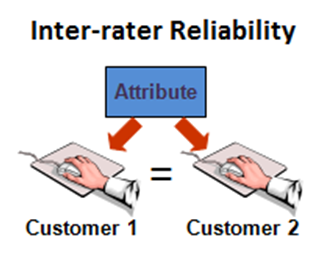### Reliability

NTRODUCTION

• Reliability of an instrument indicates the stability and consistency with which the instrument measures the concept.
• A measure is reliable when we get the same result repeatedly.
• Reliability is consistency across time (Test-retest) across items (internal consistency) and across researcher (inter ratter reliability)

DEFINITION

• The reliability of an instrument is the degree of consistency with which it measures the attribute it is supposed to be measuring.
• Reliability is the degree to which an assessment tool produces stable and consistent result.To Extend to which a measure is free of random measurement error.

Example-Weighing scale giving different results every time can’t be reliableTHE CONCEPT OF RELIABILITY

• The greater the degree of consistency and stability in a research instrument, the greater the reliability
• A scale or test is reliable to the extent that repeat measurements made by it under constant conditions will give the same result

FACTORS INFLUENCING THE RELIABILITY

• INTRINSIC FACTORS
• EXTRINSIC FACTORS

INTRINSIC FACTORS

1. LENGTH OF THE TEST
2. HOMOGENEITY OF ITEMS
3. DIFFICULTY VALUE OF ITEMS
4. DISCRIMINATIVE VALUE
5. TEST INSTRUCTION
6. ITEM SELECTION
7. RELIABILITY OF THE SCORER

EXTRINSIC FACTORS

1. GROUP VARIABILITY
2. GUESSING & CHANCE ERROR
3. ENVIRONMENTAL CONDITIONS
4. MOMENTARY FLUCTUATIONS

TYPES OF RELIABILITY

1. Stability reliability (Test-Retest)
2. Internal consistency reliability (split-half method)
3. Equivalence reliability (inter ratter reliability)Stability reliability (Test-Retest)

• The stability of an instrument is the extent to which similar results are obtained on repeated trials.
• In this type of reliability, the same tool is administered to a group of subjects on two separate occasions.
• Two sets of score obtained through administration of tools on two different occasions.
• To get exact reliability score researcher or statistics use reliability coefficient
• Which are measure by the use of “KARLS PEARSONS” correlation coefficient formula
• If the x is score of 1st test y is score of 2nd test then correlation coefficient score is calculated asSplit Half Reliability

• Split-half testing is a measure of internal consistency
• It tells how well the test components contribute to the construct that’s being measured
• Then the tool is administered to the subjects, the tool score of each half is computed and then correlation coefficient is calculated between two sets of score by KARL PEARSON method• This correlation coefficient only estimates the reliability of each half of the instrument. For adjusting the correlation coefficient for the entire instruments, the
• SPEARMAN-BROWN PROPHECY formula usedEQUIVALENCE (Inter-Ratter Reliability)

• Equivalence is used to the degree to which different independent observer, coders, or ratter agrees in their assessment decisions.
• A rating scale is developed to assess cleanliness of the Intensive Care unit
• This rating may be administered to observe the cleanliness of the Intensive Care unit by two different observers simultaneously but independently.• They used their different test to assess the cleanliness of the ICU
• On same group within a short period of time.
• Two or more observer rates the same subjects and then correlation coefficient is computed to show the strength of relationship between their observations.
• The mostly used statics techniques are Inter-class correlation coefficient and Rank order correlation coefficient

FACTORS TO IMPROVE RELIABILITY

• Standardization of condition under which data collection takes place
• Tapping of same concept by items
• Discriminating power of items
• Greater size of instruments
• Clarity of underlying dimension
• Precision in defining category
• Thorough training of observers
• Heterogeneity of the sample